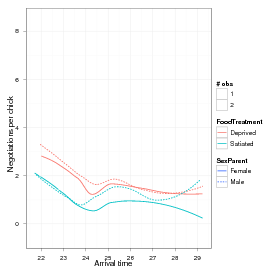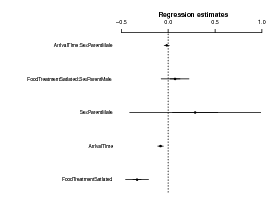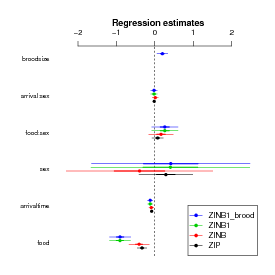## Getting started with the glmmADMB package

January 2, 2012

### 1 Introduction/quick start

glmmADMB is a package, built on the open source AD Model Builder nonlinear fitting engine, for fitting generalized linear mixed models and extensions.

• response distributions: Poisson, binomial, negative binomial (NB1 and NB2 parameterizations), Gamma, Beta, truncated Poisson and negative binomial; Gaussian coming soon
• link functions: log, logit, probit, cloglog, inverse, identity
• zero-inflation (models with a constant zero-inflation value only); hurdle models via truncated Poisson/NB
• single or multiple (nested or crossed) random effects
• offsets
• post-fit MCMC chain for characterizing uncertainty

As of version 0.6.5, the package has been greatly revised to allow a wider range of response and link functions and to allow models with multiple random effects. For now, the resulting package is slower than the old (single-random-effect version), but we hope to increase its speed in the future.

In order to use glmmADMB effectively you should already be reasonably familiar with generalized linear mixed models (GLMMs), which in turn requires familiarity with (i) generalized linear models (e.g. the special cases of logistic, binomial, and Poisson regression) and (ii) ‘modern’ mixed models (those working via maximization of the marginal likelihood rather than by manipulating sums of squares).

In order to fit a model in glmmADMB you need to:

• specify a model for the fixed effects, in the standard R (Wilkinson-Rogers) formula notation (see ?formula or Section 11.1 of the Introduction to R. Formulae can also include offsets.
• specify a model for the random effects, in the notation that is common to the nlme and lme4 packages. Random effects are specified as e|g, where e is an effect and g is a grouping factor (which must be a factor variable, or a nesting of/interaction among factor variables). For example, the formula would be 1|block for a random-intercept model or time|block for a model with random variation in slopes through time across groups specified by block. A model of nested random effects (block within site) would be 1|site/block; a model of crossed random effects (block and year) would be (1|block)+(1|year).

Random effects can be specified either in a separate random argument (as in nlme) or as part of the model formula (as in lme4).

• choose the error distribution by specifying the family (as a string: e.g. "poisson" or "binomial")
• specify a link function (as a string: e.g. "logit" or "log".
• optionally specify that zero-inflation is present zeroInflation=TRUE. In the current version, zero-inflation can only be specified as a single constant term across the entire model — i.e. it cannot vary across groups or with covariates.

### 2 Owls data

These data, taken from  and ultimately from , quantify the number of negotiations among owlets (owl chicks) in different nests prior to the arrival of a provisioning parent as a function of food treatment (deprived or satiated), the sex of the parent, and arrival time. The total number of calls from the nest is recorded, along with the total brood size, which is used as an offset to allow the use of a Poisson response.

Since the same nests are measured repeatedly, the nest is used as a random effect. The model can be expressed as a zero-inflated generalized linear mixed model (ZIGLMM).

First we draw some pictures (Figures 1, 2).

> library(ggplot2)

Various small manipulations of the data set: (1) reorder nests by mean negotiations per chick, for plotting purposes; (2) add log brood size variable (for offset); (3) rename response variable.

> Owls <- transform(Owls,
Nest=reorder(Nest,NegPerChick),
logBroodSize=log(BroodSize),
NCalls=SiblingNegotiation)Figure 1: Basic view of owl data (arrival time not shown).Figure 2: Basic view of owl data, #2 (nest identity not shown)

Now fit some models:

The basic glmmadmb fit — a zero-inflated Poisson model.

offset(logBroodSize)+(1|Nest),
data=Owls,
zeroInflation=TRUE,
family="poisson")
> summary(fit_zipoiss)
Call:
glmmadmb(formula = NCalls ~ (FoodTreatment + ArrivalTime) * SexParent +
offset(logBroodSize) + (1 | Nest), data = Owls, family = "poisson",
zeroInflation = TRUE)

Coefficients:
Estimate Std. Error z value Pr(>|z|)
(Intercept)                           2.8562     0.3871    7.38  1.6e-13 ***
FoodTreatmentSatiated                -0.3314     0.0635   -5.22  1.8e-07 ***
ArrivalTime                          -0.0807     0.0156   -5.18  2.3e-07 ***
SexParentMale                         0.2882     0.3575    0.81     0.42
FoodTreatmentSatiated:SexParentMale   0.0740     0.0761    0.97     0.33
ArrivalTime:SexParentMale            -0.0150     0.0143   -1.05     0.29
---
Signif. codes:  0 ‘***’ 0.001 ‘**’ 0.01 ‘*’ 0.05 ‘.’ 0.1 ‘ ’ 1

Number of observations: total=599, Nest=27
Random effect variance(s):
\$Nest
(Intercept)
(Intercept)     0.14001

Zero-inflation: 0.25833  (std. err.:  0.018107 )

Log-likelihood: -1985.3

> library(coefplot2)
> coefplot2(fit_zipoiss)We can also try a standard zero-inflated negative binomial model; the default is the “NB2” parameterization (variance = μ(1 + μ∕k)).

offset(logBroodSize)+(1|Nest),
data=Owls,
zeroInflation=TRUE,
family="nbinom")

Alternatively, use an “NB1” fit (variance = ϕμ).

offset(logBroodSize)+(1|Nest),
data=Owls,
zeroInflation=TRUE,
family="nbinom1")

Relax the assumption that total number of calls is strictly proportional to brood size (i.e. using log(brood size) as an offset):

BroodSize+(1|Nest),
data=Owls,
zeroInflation=TRUE,
family="nbinom1")

Every change we have made so far improves the fit — changing distributions improves it enormously, while changing the role of brood size makes only a modest (-1 AIC unit) difference:

> library(bbmle)
> AICtab(fit_zipoiss,fit_zinbinom,fit_zinbinom1,fit_zinbinom1_bs)
dAIC  df
fit_zinbinom1_bs   0.0 10
fit_zinbinom1      1.2 9
fit_zinbinom      68.7 9
fit_zipoiss      637.0 8

Compare the parameter estimates:

> vn <- c("food","arrivaltime","sex","food:sex","arrival:sex","broodsize")
> coefplot2(list(ZIP=fit_zipoiss,
ZINB=fit_zinbinom,
ZINB1=fit_zinbinom1,
ZINB1_brood=fit_zinbinom1_bs),
varnames=vn,
legend=TRUE)#### 2.1 Hurdle models

In contrast to zero-inflated models, hurdle models treat zero-count and non-zero outcomes as two completely separate categories, rather than treating the zero-count outcomes as a mixture of structural and sampling zeros.

As of version 0.6.7.1, glmmADMB includes truncated Poisson and negative binomial familes and hence can fit hurdle models. The two parts of the model have to be fitted separately, however. First we fit a truncated distribution to the non-zero outcomes:

BroodSize+(1|Nest),
data=subset(Owls,NCalls>0),
family="truncnbinom1")

Then we fit a model to the binary part of the data (zero vs. non-zero). In this case, I started by fitting a simple (intercept-only) model with intercept-level random effects only. This comes a bit closer to matching the previous (zero-inflation) models, which treated zero-inflation as a single constant level across the entire data set (in fact, leaving out the random effects and just using glmmADMB(nz~1,data=Owls,family="binomial"), or glm(nz~1,data=Owls,family="binomial"), would be an even closer match). I then fitted a more complex binary model — this is all a matter of judgment about how complex a model it’s worth trying to fit to a given data set — but it does look as though the zero-inflation varies with arrival time and satiation.

> Owls\$nz <- as.numeric(Owls\$NCalls>0)
data=Owls,
family="binomial")
data=Owls,
family="binomial")
> AICtab(fit_count,fit_ccount)
> summary(fit_ccount)

#### 2.2 MCMC fitting

AD Model Builder has the capability to run a post hoc Markov chain to assess variability — that is, it uses the MLE as a starting point and the estimated sampling distribution (variance-covariance matrix) of the parameters as a candidate distribution, and “jumps around” the parameter space in a consistent way (Metropolis-Hastings?) to generate a series of samples from a posterior distribution of the parameter distribution (assuming flat priors: please see the ADMB documentation, or , for more details).

This is very convenient, but tends to be a bit slow. In the example below, I ran a chain of 50,000 MCMC iterations — on examination, the default chain of 1000 iterations was much too short — which took about 1.92 hours on a modern (2011) laptop.

BroodSize+(1|Nest),
data=Owls,
zeroInflation=TRUE,
family="nbinom1",
mcmc=TRUE,
mcmc.opts=mcmcControl(mcmc=50000))

Convert the MCMC chain to an mcmc object which the coda package can handle:

> library(coda)
> m <- as.mcmc(fit_zinbinom1_bs_mcmc\$mcmc)

Look at the trace plots.

> library(scapeMCMC)
> plotTrace(m)The Geweke diagnostic gives Z scores for each variable for a comparison between (by default) the first 10% and last 50% of the chain

> (gg <- geweke.diag(m))

Fraction in 1st window = 0.1
Fraction in 2nd window = 0.5

pz    beta.1    beta.2    beta.3    beta.4    beta.5    beta.6    beta.7
1.05890  -0.02392   0.52422   1.26288  -1.42940  -0.10490  -0.42814   0.23393
tmpL log_alpha      u.01      u.02      u.03      u.04      u.05      u.06
0.40161  -1.64034  -0.54043  -0.10781   0.15012   0.38768  -0.21563   0.36554
u.07      u.08      u.09      u.10      u.11      u.12      u.13      u.14
0.31533   0.34659  -0.34155  -0.09860   0.15218   0.18873   0.16287   0.20951
u.15      u.16      u.17      u.18      u.19      u.20      u.21      u.22
0.16704   0.19575   0.32859   0.10631   0.13427  -0.14341   0.24088  -0.90355
u.23      u.24      u.25      u.26      u.27
0.43930   1.15159   0.34327  -0.23469   0.00186

> summary(2*pnorm(abs(gg\$z),lower.tail=FALSE))

Min. 1st Qu.  Median    Mean 3rd Qu.    Max.
0.1009  0.6685  0.8096  0.7134  0.8790  0.9985

The most frequently used diagnostic, Gelman-Rubin (gelman.diag), requires multiple chains. The full set of diagnostic functions available in coda is:

 autocorr.diag gelman.diag   geweke.diag   heidel.diag   raftery.diag

effectiveSize gives the effective length of the chain for each variable, i.e. the number of samples corrected for autocorrelation:

> range(effectiveSize(m))

 316.7461 702.1153

HPDinterval gives the highest posterior density (credible interval):

lower      upper
pz       0.04086837  0.1350379
beta.1  39.65328510 48.7512345
beta.2 -10.48568580 -6.1049157
beta.3  -8.21234181 -4.3009885
beta.4  -1.04757046  3.2400361
beta.5   0.88345874  8.9078930

You might prefer inferences based on the quantiles instead:

2.5%      97.5%
pz       0.04555855  0.1458387
beta.1  39.87602463 49.0420272
beta.2 -10.60885261 -6.1931622
beta.3  -8.46562399 -4.3939505
beta.4  -0.81607569  3.5588189
beta.5   1.55442942  9.8495245

You can also look at density plots or pairwise scatterplots (“splom” in lattice and scapeMCMC, for Scatterplot matrices), although these are not particularly useful for this large a set of parameters:

The MCMC output in glmmADMB is currently in a very raw form — in particular, the internal names and variants of the parameters are used:

pz
zero-inflation parameter
beta
fixed-effect parameter estimates: note that these are the versions of the parameters fitted internally, using an orthogonalized version of the original design matrix, not the original coefficients (if this means nothing to you, as it might well, just accept that these are transformed versions of the parameters).
tmpL
variance-covariance parameters
log_alpha
log of overdispersion/scale parameter
u
random effects (unscaled)

If you need to use the MCMC output and can’t figure out how, please contact the maintainers and encourage them to work on them some more (!)

### 3 Other information

The standard set of accessors is available:

coef
extract (fixed-effect) coefficients
fixef
a synonym for coef, for consistency with nlme/lme4
ranef
extract random effect coefficients (“BLUPs” or “conditional modes”)
residuals
extract (Pearson) residuals
fitted
fitted values
predict
predicted values (based only on fixed effects, not on random effects), possibly with standard errors (based only on uncertainty of fixed effects), possibly for new data
logLik
extract log-likelihood
AIC
extract AIC
summary
print summary
stdEr
extract standard errors of coefficients
vcov
extract estimated variance-covariance matrix of coefficients
VarCorr
extract variance-covariance matrices of random effects
confint
extract confidence intervals of fixed-effect coefficients

Missing: specifying starting values; MCMC; general troubleshooting (extra arguments, running outside R)

### References

   Benjamin M. Bolker. Ecological Models and Data in R. Princeton University Press, Princeton, NJ, 2008.

   A. Roulin and L. Bersier. Nestling barn owls beg more intensely in the presence of their mother than in the presence of their father. Animal Behaviour, 74:1099–1106, 2007.

   Alain F. Zuur, Elena N. Ieno, Neil J. Walker, Anatoly A. Saveliev, and Graham M. Smith. Mixed Effects Models and Extensions in Ecology with R. Springer, 1 edition, March 2009.